# oes() - occurrence part of iETS model

#### 2021-06-13

smooth package has a mechanism of treating the data with zero values. This might be useful for cases of intermittent demand (the demand that happens at random). All the univariate functions in the package have a parameter occurrence that allows handling this type of data. The canonical model, used in smooth, is called “iETS” - intermittent exponential smoothing model. This vignette explains how the iETS model and its occurrence part are implemented in the smooth package.

## The basics

The canonical general iETS model (called iETS$$_G$$) can be summarised as: $\begin{equation} \label{eq:iETS} \tag{1} \begin{matrix} y_t = o_t z_t \\ o_t \sim \text{Bernoulli} \left(p_t \right) \\ p_t = f(\mu_{a,t}, \mu_{b,t}) \\ a_t = w_a(v_{a,t-L}) + r_a(v_{a,t-L}) \epsilon_{a,t} \\ v_{a,t} = f_a(v_{a,t-L}) + g_a(v_{a,t-L}) \epsilon_{a,t} \\ b_t = w_a(v_{b,t-L}) + r_a(v_{b,t-L}) \epsilon_{b,t} \\ v_{b,t} = f_a(v_{b,t-L}) + g_a(v_{b,t-L}) \epsilon_{b,t} \end{matrix}, \end{equation}$ where $$y_t$$ is the observed values, $$z_t$$ is the demand size, which is a pure multiplicative ETS model on its own, $$w(\cdot)$$ is the measurement function, $$r(\cdot)$$ is the error function, $$f(\cdot)$$ is the transition function and $$g(\cdot)$$ is the persistence function (the subscripts allow separating the functions for different parts of the model). These four functions define how the elements of the vector $$v_{t}$$ interact with each other. Furthermore, $$\epsilon_{a,t}$$ and $$\epsilon_{b,t}$$ are the mutually independent error terms that follow unknown distribution, $$o_t$$ is the binary occurrence variable (1 - demand is non-zero, 0 - no demand in the period $$t$$) which is distributed according to Bernoulli with probability $$p_t$$. $$\mu_{a,t}$$ and $$\mu_{b,t}$$ are the conditional expectations for the unobservable variables $$a_t$$ and $$b_t$$. Any ETS model can be used for $$a_t$$ and $$b_t$$, and the transformation of them into the probability $$p_t$$ depends on the type of the error. The general formula for the multiplicative error is: $\begin{equation} \label{eq:oETS(MZZ)} p_t = \frac{\mu_{a,t}}{\mu_{a,t}+\mu_{b,t}} , \end{equation}$ while for the additive error it is: $\begin{equation} \label{eq:oETS(AZZ)} p_t = \frac{\exp(\mu_{a,t})}{\exp(\mu_{a,t})+\exp(\mu_{b,t})} . \end{equation}$ This is because both $$\mu_{a,t}$$ and $$\mu_{b,t}$$ need to be positive, and the additive error models support the real plane. The canonical iETS model assumes that the pure multiplicative model is used for the both $$a_t$$ and $$b_t$$. This type of model is positively defined for any values of error, trend and seasonality, which is essential for the values of $$a_t$$ and $$b_t$$ and their expectations. If a combination of additive and multiplicative error models is used, then the additive part is exponentiated prior to the usage of the formulae for the calculation of the probability.

An example of an iETS model is the basic local-level model iETS(M,N,N)$$_G$$(M,N,N)(M,N,N): $\begin{equation} \label{eq:iETSGExample} \begin{matrix} y_t = o_t z_t \\ z_t = l_{z,t-1} \left(1 + \epsilon_{z,t} \right) \\ l_{z,t} = l_{z,t-1}( 1 + \alpha_{z} \epsilon_{z,t}) \\ (1 + \epsilon_{t}) \sim \text{log}\mathcal{N}(0, \sigma_\epsilon^2) \\ \\ o_t \sim \text{Bernoulli} \left(p_t \right) \\ p_t = \frac{\mu_{a,t}}{\mu_{a,t}+\mu_{b,t}} \\ \\ a_t = l_{a,t-1} \left(1 + \epsilon_{a,t} \right) \\ l_{a,t} = l_{a,t-1}( 1 + \alpha_{a} \epsilon_{a,t}) \\ \mu_{a,t} = l_{a,t-1} \\ \\ b_t = l_{b,t-1} \left(1 + \epsilon_{b,t} \right) \\ l_{b,t} = l_{b,t-1}( 1 + \alpha_{b} \epsilon_{b,t}) \\ \mu_{b,t} = l_{b,t-1} \\ \end{matrix}, \end{equation}$ where $$l_{a,t}$$ and $$l_{b,t}$$ are the levels for each of the shape parameters and $$\alpha_{a}$$ and $$\alpha_{b}$$ are the smoothing parameters and the error terms $$1+\epsilon_{a,t}$$ and $$1+\epsilon_{b,t}$$ are positive and have means of one. We do not make any other distributional assumptions concerning the error terms. More advanced models can be constructing by specifying the ETS models for each part and / or adding explanatory variables.

In the notation of the model iETS(M,N,N)$$_G$$(M,N,N)(M,N,N), the first brackets describe the ETS model for the demand sizes, the underscore letter points out at the specific subtype of model (see below), the second brackets describe the ETS model, underlying the variable $$a_t$$ and the last ones stand for the model for the $$b_t$$. If only one variable is needed (either $$a_t$$ or $$b_t$$), then the redundant brackets are dropped, so that the notation simplifies, for example, to: iETS(M,N,N)$$_O$$(M,N,N). If the same type of model is used for both demand sizes and demand occurrence, then the second brackets can be dropped as well, simplifying the view to: iETS(M,N,N)$$_G$$. Furthermore, the notation without any brackets, such as iETS$$_G$$ stands for a general class of a specific subtype of iETS model (so any error / trend / seasonality). Also, given that iETS$$_G$$ is the most general model of all iETS models, the “$$G$$” can be dropped, when the properties are applicable to all subtypes. Finally, the “oETS” notation is used when the occurrence part of the model is discussed explicitly, skipping the demand sizes.

The concentrated likelihood function for the iETS model is: $\begin{equation} \label{eq:LogNormalConcentratedLogLikelihood} \tag{2} \ell(\boldsymbol{\theta}, \hat{\sigma}_\epsilon^2 | \textbf{Y}) = - \frac{1}{2} \left( T \log(2 \pi e \hat{\sigma}_\epsilon^2) + T_0 \right) - {\sum_{o_t=1}} \log(z_t) + {\sum_{o_t=1}} \log(\hat{p}_t) + {\sum_{o_t=0}} \log(1-\hat{p}_t) , \end{equation}$ where $$\textbf{Y}$$ is the vector of all the in-sample observations, $$\boldsymbol{\theta}$$ is the vector of parameters to estimate (initial values and smoothing parameters), $$T$$ is the number of all observations, $$T_0$$ is the number of zero observations, $$\hat{\sigma_\epsilon}^2 = \frac{1}{T} \sum_{o_t=1} \log^2 \left(1 + \epsilon_t \right)$$ is the scale parameter of the one-step-ahead forecast error for the demand sizes and $$\hat{p}_t$$ is the estimated probability of a non-zero demand at time $$t$$. This likelihood is used for the estimation of all the special cases of the iETS$$_G$$ model.

Depending on the restrictions on $$a_t$$ and $$b_t$$, there can be several iETS models:

1. iETS$$_F$$: $$\mu_{a,t} = \text{const}$$, $$\mu_{b,t} = \text{const}$$ - the model with the fixed probability of occurrence;
2. iETS$$_O$$: $$\mu_{b,t} = 1$$ - the “odds ratio” model, based on the logistic transform of the $$o_t$$ variable;
3. iETS$$_I$$: $$\mu_{a,t} = 1$$ - the “inverse odds ratio” model, based on the inverse logistic transform of the $$o_t$$ variable;
4. iETS$$_D$$: $$\mu_{a,t} + \mu_{b,t} = 1$$, $$\mu_{a,t} \leq 1$$ - the direct probability model, where the $$p_t$$ is calculated directly from the occurrence variable $$o_t$$;
5. iETS$$_G$$: No restrictions - the model based on the evolution of both $$\mu_{a,t}$$ and $$\mu_{b,t}$$.

Depending on the type of the model, there are different mechanisms of the model construction, error calculation, update of the states and the generation of forecasts.

In this vignette we will use ETS(M,N,N) model as a base for the different parts of the models. Although, this is a simplification, it allows better understanding the basics of the different types of iETS model, without the loss of generality.

We will use an artificial data in order to see how the functions work:

y <- ts(c(rpois(20,0.25),rpois(20,0.5),rpois(20,1),rpois(20,2),rpois(20,3),rpois(20,5)))

All the models, discussed in this vignette, are implemented in the functions oes() and oesg(). The only missing element in all of this at the moment is the model selection mechanism for the demand occurrence part. So neither oes() nor oesg() currently support “ZZZ” ETS models.

## iETS$$_F$$

In case of the fixed $$a_t$$ and $$b_t$$, the iETS$$_G$$ model reduces to: $\begin{equation} \label{eq:ISSETS(MNN)Fixed} \tag{3} \begin{matrix} y_t = o_t z_t \\ o_t \sim \text{Bernoulli}(p) \end{matrix} . \end{equation}$

The conditional h-steps ahead mean of the demand occurrence probability is calculated as: $\begin{equation} \label{eq:pt_fixed_expectation} \hat{o}_{t+h|t} = \hat{p} . \end{equation}$

The estimate of the probability $$p$$ is calculated based on the maximisation of the following concentrated log-likelihood function: $\begin{equation} \label{eq:ISSETS(MNN)FixedLikelihoodSmooth} \ell \left({p} | o_t \right) = T_1 \log {p} + T_0 \log (1-{p}) , \end{equation}$ where $$T_0$$ is the number of zero observations and $$T_1$$ is the number of non-zero observations in the data. The number of estimated parameters in this case is equal to $$k_z+1$$, where $$k_z$$ is the number of parameters for the demand sizes part, and 1 is for the estimation of the probability $$p$$. Maximising this likelihood deems the analytical solution for the $$p$$: $\begin{equation} \label{eq:ISSETS(MNN)FixedLikelihoodSmoothProbability} \hat{p} = \frac{T_1}{T}, \end{equation}$ where $$T_1$$ is the number of non-zero observations and $$T$$ is the number of all the available observations.

The occurrence part of the model oETS$$_F$$ is constructed using oes() function:

oETSFModel1 <- oes(y, occurrence="fixed", h=10, holdout=TRUE)
oETSFModel1
## Occurrence state space model estimated: Fixed probability
## Underlying ETS model: oETS[F](MNN)
## Vector of initials:
##  level
## 0.6455
##
## Error standard deviation: 1.0909
## Sample size: 110
## Number of estimated parameters: 1
## Number of degrees of freedom: 109
## Information criteria:
##      AIC     AICc      BIC     BICc
## 145.0473 145.0844 147.7478 147.8349
plot(oETSFModel1)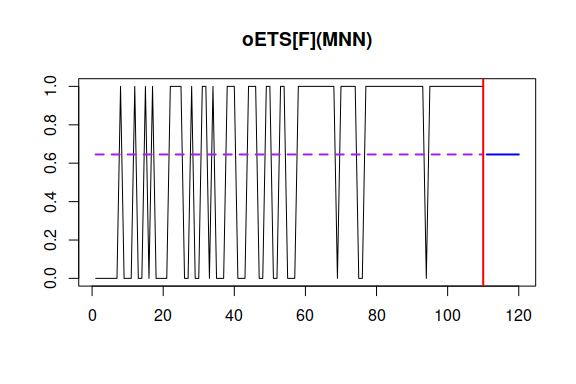The occurrence part of the model is supported by adam() function. For example, here’s how the iETS(M,M,N)$$_F$$ can be constructed:

adam(y, "MMN", occurrence="fixed", h=10, holdout=TRUE, silent=FALSE)
## Time elapsed: 0.02 seconds
## Model estimated using adam() function: iETS(MMN)[F]
## Occurrence model type: Fixed probability
## Distribution assumed in the model: Mixture of Bernoulli and Gamma
## Loss function type: likelihood; Loss function value: -1.112383e+39
## Persistence vector g:
##  alpha   beta
## 0.6026 0.5127
##
## Sample size: 110
## Number of estimated parameters: 6
## Number of degrees of freedom: 104
## Information criteria:
##           AIC          AICc           BIC          BICc
## -2.224767e+39 -2.224767e+39 -2.224767e+39 -2.224767e+39
##
## Forecast errors:
## Bias: -100%; sMSE: Inf%; rRMSE: Inf; sPIS: 2.33930983218327e+280%; sCE: -2.33582780103154e+280%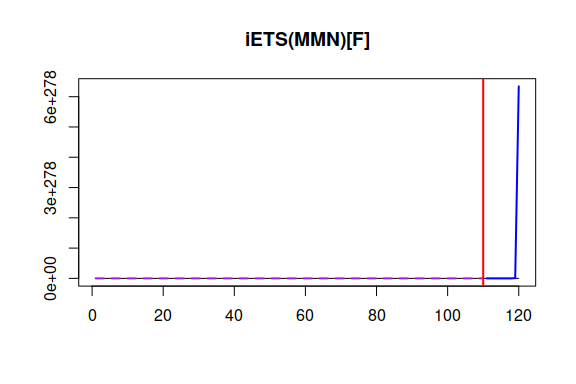## iETS$$_O$$

The odds-ratio iETS uses only one model for the occurrence part, for the $$\mu_{a,t}$$ variable (setting $$\mu_{b,t}=1$$), which simplifies the iETS$$_G$$ model. For example, for the iETS$$_O$$(M,N,N): $\begin{equation} \label{eq:iETSO} \tag{5} \begin{matrix} y_t = o_t z_t \\ o_t \sim \text{Bernoulli} \left(p_t \right) \\ p_t = \frac{\mu_{a,t}}{\mu_{a,t}+1} \\ a_t = l_{a,t-1} \left(1 + \epsilon_{a,t} \right) \\ l_{a,t} = l_{a,t-1}( 1 + \alpha_{a} \epsilon_{a,t}) \\ \mu_{a,t} = l_{a,t-1} \end{matrix}. \end{equation}$

In the estimation of the model, the initial level is set to the transformed mean probability of occurrence $$l_{a,0}=\frac{\bar{p}}{1-\bar{p}}$$ for multiplicative error model and $$l_{a,0} = \log l_{a,0}$$ for the additive one, where $$\bar{p}=\frac{1}{T} \sum_{t=1}^T o_t$$, the initial trend is equal to 0 in case of the additive and 1 in case of the multiplicative types. In cases of seasonal models, the regression with dummy variables is fitted, and its parameters are then used for the initials of the seasonal indices after the transformations similar to the level ones.

The construction of the model is done via the following set of equations (example with oETS$$_O$$(M,N,N)): $\begin{equation} \label{eq:iETSOEstimation} \begin{matrix} \hat{p}_t = \frac{\hat{a}_t}{\hat{a}_t+1} \\ \hat{a}_t = l_{a,t-1} \\ l_{a,t} = l_{a,t-1}( 1 + \alpha_{a} e_{a,t}) \\ 1+e_{a,t} = \frac{u_t}{1-u_t} \\ u_{t} = \frac{1 + o_t - \hat{p}_t}{2} \end{matrix}, \end{equation}$ where $$\hat{a}_t$$ is the estimate of $$\mu_{a,t}$$.

Given that the model is estimated using the likelihood (2), it has $$k_z+k_a$$ parameters to estimate, where $$k_z$$ includes all the initial values, the smoothing parameters and the scale of the error of the demand sizes part of the model, and $$k_a$$ includes only initial values and the smoothing parameters of the model for the demand occurrence. In case of iETS$$_O$$(M,N,N) this number is equal to 5.

The occurrence part of the model iETS$$_O$$ is constructed using the very same oes() function, but also allows specifying the ETS model to use. For example, here’s the ETS(M,M,N) model:

oETSOModel <- oes(y, model="MMN", occurrence="o", h=10, holdout=TRUE)
oETSOModel
## Occurrence state space model estimated: Odds ratio
## Underlying ETS model: oETS[O](MMN)
## Smoothing parameters:
##  level  trend
## 0.0127 0.0005
## Vector of initials:
##  level  trend
## 0.2001 1.0163
##
## Error standard deviation: 1.8066
## Sample size: 110
## Number of estimated parameters: 4
## Number of degrees of freedom: 106
## Information criteria:
##      AIC     AICc      BIC     BICc
## 113.8571 114.2381 124.6591 125.5544
plot(oETSOModel)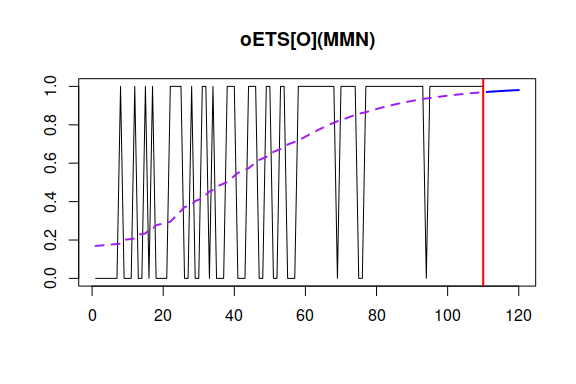And here’s the full iETS(M,M,N)$$_O$$ model:

adam(y, "MMN", occurrence="o", oesmodel="MMN", h=10, holdout=TRUE, silent=FALSE)
## Time elapsed: 0.07 seconds
## Model estimated using adam() function: iETS(MMN)[O]
## Occurrence model type: Odds ratio
## Distribution assumed in the model: Mixture of Bernoulli and Gamma
## Loss function type: likelihood; Loss function value: -1.112383e+39
## Persistence vector g:
##  alpha   beta
## 0.6026 0.5127
##
## Sample size: 110
## Number of estimated parameters: 9
## Number of degrees of freedom: 101
## Information criteria:
##           AIC          AICc           BIC          BICc
## -2.224767e+39 -2.224767e+39 -2.224767e+39 -2.224767e+39
##
## Forecast errors:
## Bias: -100%; sMSE: Inf%; rRMSE: Inf; sPIS: 3.55520905240429e+280%; sCE: -3.54992208492897e+280%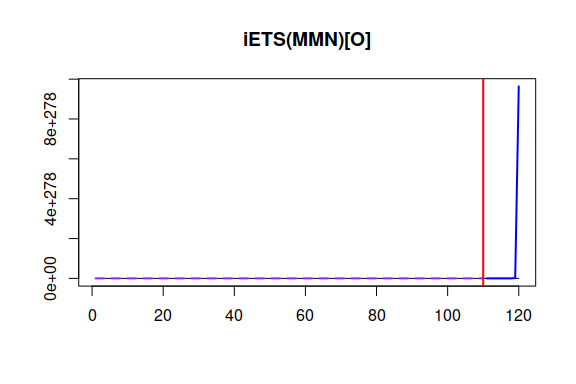This should give the same results as before, meaning that we ask explicitly for the adam() function to use the earlier estimated model:

adam(y, "MMN", occurrence=oETSOModel, h=10, holdout=TRUE, silent=FALSE)

This gives an additional flexibility, because the construction can be done in two steps, with a more refined model for the occurrence part (e.g. including explanatory variables).

## iETS$$_I$$

Similarly to the odds-ratio iETS, inverse-odds-ratio model uses only one model for the occurrence part, but for the $$\mu_{b,t}$$ variable instead of $$\mu_{a,t}$$ (now $$\mu_{a,t}=1$$). Here is an example of iETS$$_I$$(M,N,N): $\begin{equation} \label{eq:iETSI} \tag{6} \begin{matrix} y_t = o_t z_t \\ o_t \sim \text{Bernoulli} \left(p_t \right) \\ p_t = \frac{1}{1+\mu_{b,t}} \\ b_t = l_{b,t-1} \left(1 + \epsilon_{b,t} \right) \\ l_{b,t} = l_{b,t-1}( 1 + \alpha_{b} \epsilon_{b,t}) \\ \mu_{b,t} = l_{b,t-1} \end{matrix}. \end{equation}$

This model resembles the logistic regression, where the probability is obtained from an underlying regression model of $$x_t'A$$. In the estimation of the model, the initial level is set to the transformed mean probability of occurrence $$l_{b,0}=\frac{1-\bar{p}}{\bar{p}}$$ for multiplicative error model and $$l_{b,0} = \log l_{b,0}$$ for the additive one, where $$\bar{p}=\frac{1}{T} \sum_{t=1}^T o_t$$, the initial trend is equal to 0 in case of the additive and 1 in case of the multiplicative types. The seasonality is treated similar to the iETS$$_O$$ model, but using the inverse-odds transformation.

The construction of the model is done via the set of equations similar to the ones for the iETS$$_O$$ model: $\begin{equation} \label{eq:iETSIEstimation} \begin{matrix} \hat{p}_t = \frac{1}{1+\hat{b}_t} \\ \hat{b}_t = l_{b,t-1} \\ l_{b,t} = l_{b,t-1}( 1 + \alpha_{b} e_{b,t}) \\ 1+e_{b,t} = \frac{1-u_t}{u_t} \\ u_{t} = \frac{1 + o_t - \hat{p}_t}{2} \end{matrix}, \end{equation}$ where $$\hat{b}_t$$ is the estimate of $$\mu_{b,t}$$.

So the model iETS$$_I$$ is like a mirror reflection of the model iETS$$_O$$. However, it produces different forecasts, because it focuses on the probability of non-occurrence, rather than the probability of occurrence. Interestingly enough, the probability of occurrence $$p_t$$ can also be estimated if $$1+b_t$$ in the denominator is set to be equal to the demand intervals (between the demand occurrences). The model (6) underlies Croston’s method in this case.

Once again oes() function is used in the construction of the model:

oETSIModel <- oes(y, model="MMN", occurrence="i", h=10, holdout=TRUE)
oETSIModel
## Occurrence state space model estimated: Inverse odds ratio
## Underlying ETS model: oETS[I](MMN)
## Smoothing parameters:
##  level  trend
## 0.0478 0.0000
## Vector of initials:
##   level   trend
## 11.0468  0.9093
##
## Error standard deviation: 4.8583
## Sample size: 110
## Number of estimated parameters: 4
## Number of degrees of freedom: 106
## Information criteria:
##      AIC     AICc      BIC     BICc
## 116.7323 117.1133 127.5342 128.4296
plot(oETSIModel)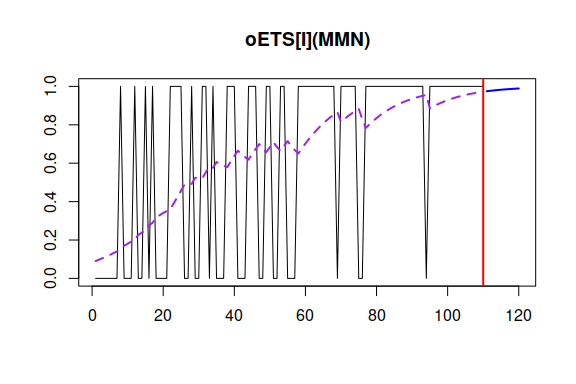And here’s the full iETS(M,M,N)$$_O$$ model:

adam(y, "MMN", occurrence="i", oesmodel="MMN", h=10, holdout=TRUE, silent=FALSE)
## Time elapsed: 0.07 seconds
## Model estimated using adam() function: iETS(MMN)
## Occurrence model type: Inverse odds ratio
## Distribution assumed in the model: Mixture of Bernoulli and Gamma
## Loss function type: likelihood; Loss function value: -1.112383e+39
## Persistence vector g:
##  alpha   beta
## 0.6026 0.5127
##
## Sample size: 110
## Number of estimated parameters: 9
## Number of degrees of freedom: 101
## Information criteria:
##           AIC          AICc           BIC          BICc
## -2.224767e+39 -2.224767e+39 -2.224767e+39 -2.224767e+39
##
## Forecast errors:
## Bias: -100%; sMSE: Inf%; rRMSE: Inf; sPIS: 3.58489171354356e+280%; sCE: -3.57956142686759e+280%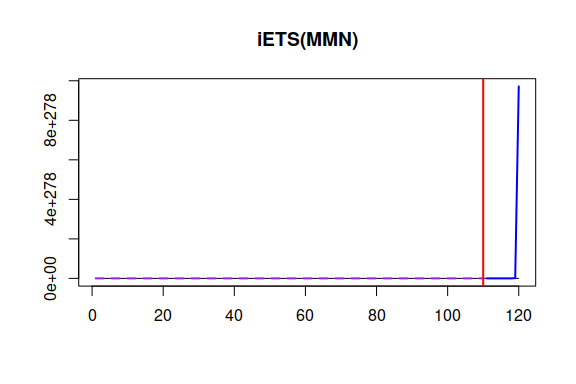Once again, an earlier estimated model can be used in the univariate forecasting functions:

adam(y, "MMN", occurrence=oETSIModel, h=10, holdout=TRUE, silent=FALSE)

## iETS$$_D$$

This model appears, when a specific restriction is imposed: $\begin{equation} \label{eq:iETSGRestriction} \mu_{a,t} + \mu_{b,t} = 1, \mu_{a,t} \in [0, 1] \end{equation}$ The pure multiplicative iETS$$_G$$(M,N,N) model is then transformed into iETS$$_D$$(M,N,N): $\begin{equation} \label{eq:iETSD} \tag{7} \begin{matrix} y_t = o_t z_t \\ o_t \sim \text{Bernoulli} \left(a_t \right) \\ a_t = l_{a,t-1} \left(1 + \epsilon_{a,t} \right) \\ l_{a,t} = l_{a,t-1}( 1 + \alpha_{a} \epsilon_{a,t}) \\ \mu_{a,t} = \min(l_{a,t-1}, 1) \end{matrix}. \end{equation}$ An option with the additive model in this case has a different, more complicated form: $\begin{equation} \label{eq:iETSDAdditive} \begin{matrix} y_t = o_t z_t \\ o_t \sim \text{Bernoulli} \left(a_t \right) \\ a_t = l_{a,t-1} + \epsilon_{a,t} \\ l_{a,t} = l_{a,t-1} + \alpha_{a} \epsilon_{a,t} \\ \mu_{a,t} = \max \left( \min(l_{a,t-1}, 1), 0 \right) \end{matrix}. \end{equation}$

The estimation of the multiplicative error model is done using the following set of equations: $\begin{equation} \label{eq:ISSETS(MNN)_probability_estimate} \begin{matrix} \hat{y}_t = o_t \hat{l}_{z,t-1} \\ \hat{l}_{z,t} = \hat{l}_{z,t-1}( 1 + \alpha e_t) \\ \hat{a}_t = min(\hat{l}_{a,t-1}, 1) \\ \hat{l}_{a,t} = \hat{l}_{a,t-1}( 1 + \alpha_{a} e_{a,t}) \end{matrix}, \end{equation}$ where $\begin{equation} \label{eq:ISSETS(MNN)_TSB_model_error_approximation} e_{a,t} = \frac{o_t (1 - 2 \kappa) + \kappa - \hat{a}_t}{\hat{a}_t}, \end{equation}$ and $$\kappa$$ is a very small number (for example, $$\kappa = 10^{-10}$$), needed only in order to make the model estimable. The estimate of the error term in case of the additive model is much simpler and does not need any specific tricks to work: $\begin{equation} \label{eq:ISSETS(MNN)_TSB_model_error_approximation2} e_{a,t} = o_t - \hat{a}_t . \end{equation}$

The initials of the iETS$$_D$$ model are calculated directly from the data without any additional transformations

Here’s an example of the application of the model to the same artificial data:

oETSDModel <- oes(y, model="MMN", occurrence="d", h=10, holdout=TRUE)
oETSDModel
## Occurrence state space model estimated: Direct probability
## Underlying ETS model: oETS[D](MMN)
## Smoothing parameters:
##  level  trend
## 0.0429 0.0000
## Vector of initials:
##  level  trend
## 0.3056 1.0084
##
## Error standard deviation: 1.0405
## Sample size: 110
## Number of estimated parameters: 4
## Number of degrees of freedom: 106
## Information criteria:
##      AIC     AICc      BIC     BICc
## 119.7640 120.1450 130.5659 131.4613
plot(oETSDModel)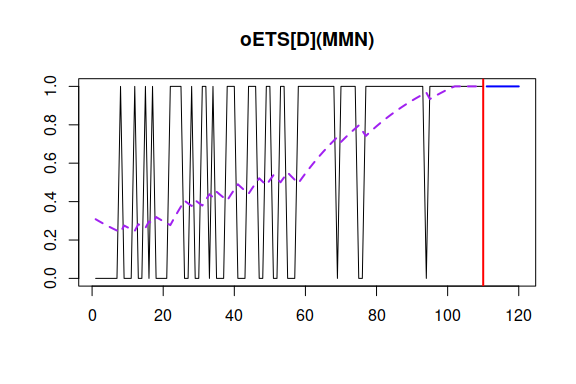The usage of the model in case of univariate forecasting functions is the same as in the cases of other occurrence models, discussed above:

adam(y, "MMN", occurrence=oETSDModel, h=10, holdout=TRUE, silent=FALSE)
## Time elapsed: 0.02 seconds
## Model estimated using adam() function: iETS(MMN)[D]
## Occurrence model type: Direct
## Distribution assumed in the model: Mixture of Bernoulli and Gamma
## Loss function type: likelihood; Loss function value: -1.112383e+39
## Persistence vector g:
##  alpha   beta
## 0.6026 0.5127
##
## Sample size: 110
## Number of estimated parameters: 5
## Number of degrees of freedom: 105
## Number of provided parameters: 4
## Information criteria:
##           AIC          AICc           BIC          BICc
## -2.224767e+39 -2.224767e+39 -2.224767e+39 -2.224767e+39
##
## Forecast errors:
## Bias: -100%; sMSE: Inf%; rRMSE: Inf; sPIS: 3.62428283859379e+280%; sCE: -3.61888814244323e+280%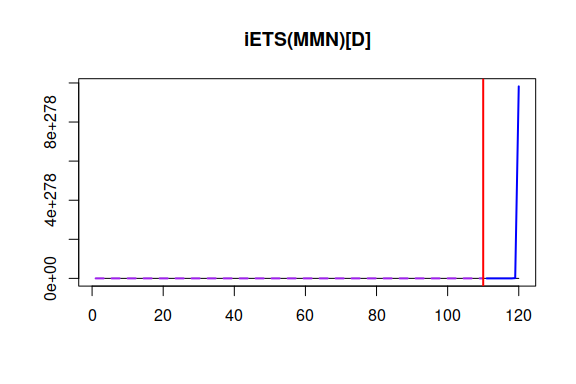## iETS$$_G$$

This model has already been discussed above and was presented in (1). The estimation of iETS(M,N,N)$$_G$$ model is done via the following set of equations: $\begin{equation} \label{eq:ISSETS(MNN)Estimated} \begin{matrix} \hat{y}_t = o_t \hat{z}_t \\ e_t = o_t \frac{y_t - \hat{z}_t}{\hat{z}_t} \\ \hat{z}_t = \hat{l}_{z,t-1} \\ \hat{l}_{z,t} = \hat{l}_{z,t-1}( 1 + \alpha_z e_t) \\ e_{a,t} = \frac{u_t}{1-u_t} -1 \\ \hat{a}_t = \hat{l}_{a,t-1} \\ \hat{l}_{a,t} = \hat{l}_{a,t-1}( 1 + \alpha_{a} e_{a,t}) \\ e_{b,t} = \frac{1-u_t}{u_t} -1 \\ \hat{b}_t = \hat{l}_{b,t-1} \\ \hat{l}_{b,t} = \hat{l}_{b,t-1}( 1 + \alpha_{b} e_{b,t}) \end{matrix} . \end{equation}$ The initialisation of the parameters of the iETS$$_G$$ model is done separately for the variables $$a_t$$ and $$b_t$$, based on the principles, described above for the iETS$$_O$$ and iETS$$_I$$.

There is a separate function for this model, called oesg(). It has twice more parameters than oes(), because it allows fine tuning of the models for the variables $$a_t$$ and $$b_t$$. This gives an additional flexibility. For example, here is how we can use ETS(M,N,N) for the $$a_t$$ and ETS(A,A,N) for the $$b_t$$, resulting in oETS$$_G$$(M,N,N)(A,A,N):

oETSGModel1 <- oesg(y, modelA="MNN", modelB="AAN", h=10, holdout=TRUE)
oETSGModel1
## Occurrence state space model estimated: General
## Underlying ETS model: oETS[G](MNN)(AAN)
##
## Sample size: 110
## Number of estimated parameters: 6
## Number of degrees of freedom: 104
## Information criteria:
##      AIC     AICc      BIC     BICc
## 120.3770 121.1926 136.5799 138.4966
plot(oETSGModel1)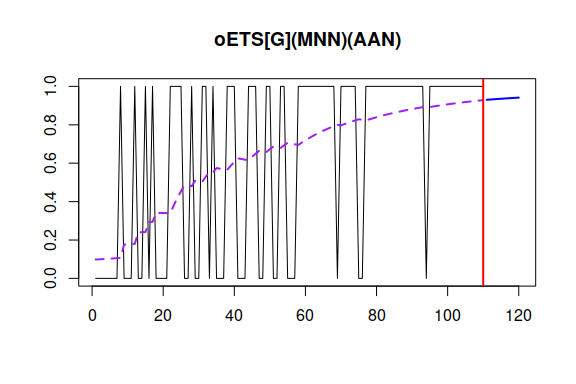The oes() function accepts occurrence="g" and in this case calls for oesg() with the same types of ETS models for both parts:

oETSGModel2 <- oes(y, model="MNN", occurrence="g", h=10, holdout=TRUE)
oETSGModel2
## Occurrence state space model estimated: General
## Underlying ETS model: oETS[G](MNN)(MNN)
##
## Sample size: 110
## Number of estimated parameters: 4
## Number of degrees of freedom: 106
## Information criteria:
##      AIC     AICc      BIC     BICc
## 119.2524 119.6334 130.0543 130.9497
plot(oETSGModel2)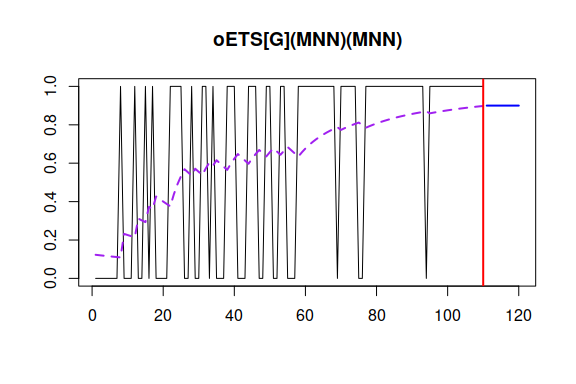Finally, the more flexible way to construct iETS model would be to do it in two steps: either using oesg() or oes() and then using the es() with the provided model in occurrence variable. But a simpler option is available as well:

es(y, "MMN", occurrence="g", oesmodel="MMN", h=10, holdout=TRUE, silent=FALSE)
## Time elapsed: 0.04 seconds
## Model estimated: ETS(AAN)
## Persistence vector g:
##  alpha   beta
## 0.0053 0.0053
## Initial values were optimised.
##
## Loss function type: likelihood; Loss function value: 203.1161
## Error standard deviation: 1.5696
## Sample size: 110
## Number of estimated parameters: 5
## Number of degrees of freedom: 105
## Information criteria:
##      AIC     AICc      BIC     BICc
## 416.2323 416.8092 429.7347 431.0906
##
## Forecast errors:
## MPE: -14.8%; sCE: 61.4%; Bias: 18%; MAPE: 38.7%
## MASE: 1.061; sMAE: 89.9%; sMSE: 48.6%; rMAE: 0.343; rRMSE: 0.381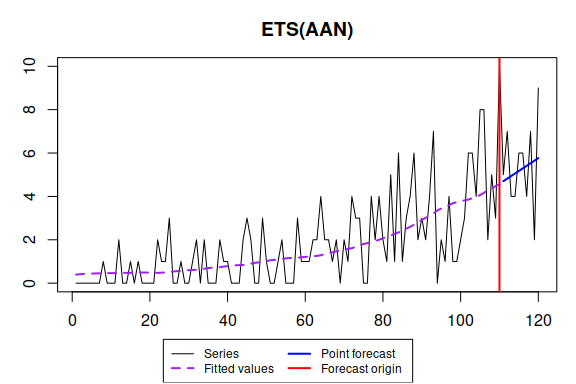## iETS$$_A$$

Finally, there is an occurrence type selection mechanism. It tries out all the iETS subtypes of models, discussed above and selects the one that has the lowest information criterion (i.e. AIC). This subtype is called iETS$$_A$$ (automatic), although it does not represent any specific model. Here’s an example:

oETSAModel <- oes(y, model="MNN", occurrence="a", h=10, holdout=TRUE)
oETSAModel
## Occurrence state space model estimated: Odds ratio
## Underlying ETS model: oETS[O](MNN)
## Smoothing parameters:
##  level
## 0.0895
## Vector of initials:
##  level
## 0.1402
##
## Error standard deviation: 1.9868
## Sample size: 110
## Number of estimated parameters: 2
## Number of degrees of freedom: 108
## Information criteria:
##      AIC     AICc      BIC     BICc
## 115.2524 115.3646 120.6534 120.9169
plot(oETSAModel)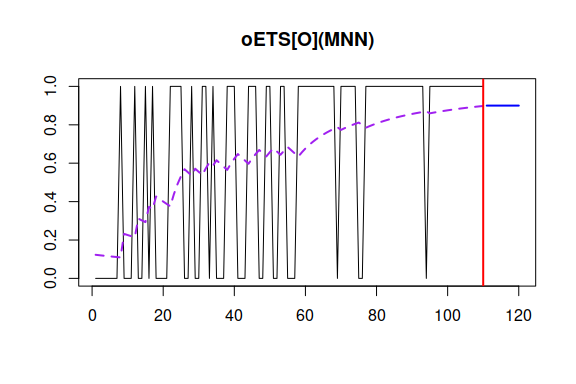The main restriction of the iETS models at the moment (smooth v.2.5.0) is that there is no model selection between the ETS models for the occurrence part. This needs to be done manually. Hopefully, this feature will appear in the next release of the package.